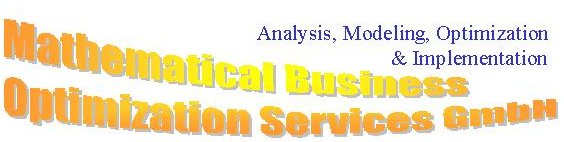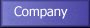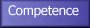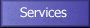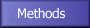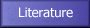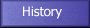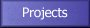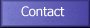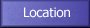#### Mixed Integer Linear Programming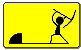Restricting the domain of all or of a part of variables xi of an LP problem to integer values an integer (ILP) or a mixed-integer linear programming problem (MILP) results.

#### Some Remarks on MILP Models

Building mixed-integer models requires great caution. Often there exist different possibilities to formulate an optimization problem, sometimes adding redundant constraints makes an algorithm work faster, e.g., if the gap between the optimal solutions of the LP-relaxation and of the original problem is diminished by this. Even some nonlinear optimization problems can be transformed to MILP's using special types of discrete variables.

• Logical conditions, such as ''and'', ''or'', ''not'', ''implies'', and also disjunctive constraints are formulated with binary variables d Î {0,1}.

• Binary variables can indicate the state of a continuous variable and at the same time impose upper and lower bounds (L and U) on this variable. The constraints x = 0ÚL Ł x Ł U defining a semi-continuous variables x are equivalent to L·d Ł x Łd, where d is a binary variable. Some software packages offer semi-continuous variables to formulate this constraint directly without utilizing an additional binary variable which provides great advantages for the B&B procedure.

• Special Ordered Sets (SOS) have been developed to formulate common types of restrictions in mathematical programming. In SOS of type 1 variables exactly one variable (continuous or integer) must be non-zero. In an SOS of type 2 set two variables which are adjacent in the ordering of the set or one single variable must be non-zero. Such sets often are used to model piecewise linear functions, e.g., linear approximations of nonlinear functions.

• Problems with products of k binary variables dp = Ői = 1kdidi Î {0,1} can be transformed directly into integer models according to
 dp Ł dj, j = 1,...,k    ;    ĺj = 1kdj-dp Ł k-1    ;    dj Î {0,1}    .

## Solution Approaches for MILP Problems

A great variety of algorithms to solve mixed integer optimization problems has arisen during the last decades. Among the best known exact algorithms for solving MILP's are the following methods:

Efficient implicit enumerative algorithms include pruning criteria so that not all feasible solutions have to be tested for finding the optimal solution and for proving optimality. The widely used Branch&Bound (B&B) algorithm with LP-relaxation (see figure ''Branch&Bound'') is the most important representative of enumerative algorithms and therefore discussed in more detail in the next subsection.

Cutting plane algorithms and Branch&Cut [Gomory (1958), Crowder et al. (1983), Wolsey and Van Roy (1987), Padberg and Rinaldi (1991), Balas et al. (1993), Boyd (1994)] for MILP's are derived from the Simplex algorithm. After computing the continuous optimum by LP-relaxation of the integrality constraints step by step new constraints are added to the MILP. With the help of these additional inequalities non integer variables of the continuous solutions are forced to take integer values. Cutting plane methods are not restricted to MILP's, they are used, e.g., in nonlinear and nondifferentiable optimization as well (Lemaréchal in ).

Dynamic programming is not a general-purpose algorithm like methods belonging to the first two groups. Originally, it was developed for the optimization of sequential decision processes. This technique for multistage problem solving may be applied to linear and nonlinear OPs which can be described as a nested family of subproblems. The original problem is solved recursively from the solutions of the subproblems.

Decomposition methods [Benders (1962), Fisher (1981), Geoffrion and McBride (1978), Magnanti and Wong (1981), van Roy (1983), Van Roy (1986)].

Logic-based methods [Balas (1975, 1979), Jeroslow (1977), Hooker (1988), Jeroslow and Lowe (1984, 1985), Jeroslow and Wang (1990), Raman and Grossmann (1992)].

Furthermore there exist heuristics, local and global search algorithms as for instance simulated annealing.

## Branch&Bound Algorithm

The first B&B algorithm was developed in 1960 by Land and Doig. The branch in B&B hints at the partitioning process -a ''divide-and-conquer'' like approach- used to prove optimality of a solution. Lower2 bounds are used during this process to avoid an exhaustive search in the solution space. The B&B idea or implicit enumeration characterizes a wide class of algorithms which can be applied to discrete optimization problems in general.

The computational steps of the B&B algorithm are: After some initialization the LP relaxation -that is that LP problem which results if we relax all integer variables to continuous one- establishes the first node. The node selection is obvious in the first step (just take the LP relaxation), later on it is based on some heuristics. A B&B algorithm of Dakin (1965) with LP relaxations uses three pruning criteria: infeasibility, optimality and value dominance relation. In a maximization problem the integer solution found lead to an increasing sequence of lower bounds while the LP problems in the tree decrease the upper bound. Note that a denotes an addcut which causes the algorithm to accept a new integer solution only if it better by at least the value of a. If the pruning criteria fail branching starts: The branching in this algorithm is done by variable dichotomy: for a fractional yj* two son nodes are created with the additional constraint yj Ł [yj*] resp. yj ³ [yj*]+1. Other possibilities for dividing the search space are for instance generalized upper bound dichotomy or enumeration of all possible values, if the domain of a variable is finite. The advantage of variable dichotomy is that only simple constraints on lower and upper bounds are added to the problem.

The search strategy plays an important role in implicit enumeration, widely used is the depth-first plus backtracking rule as presented above. If a node is not pruned, one of its two sons is considered. If a node is pruned, the algorithm goes back to the last node with a son which has not yet been considered (backtracking). In linear programming only lower and upper bound constraints are added, the dual simplex algorithm can reoptimize the problem directly without data transfer or basis reinversion. Furthermore, it is more likely that feasible solutions are found deep in the tree as experience has shown. Nevertheless, in some cases the use of the opposite strategy, breadth-first search, may be advantageous.

Another important point is the selection of the branching variable. A common way of choosing a branching variable is by user-specified priorities, because no robust general strategy is known. Degradations or penalties may also be used to choose the branching variables, both methods estimate or calculate the increase of the objective function value if a variable is required to be integral, especially penalties are costly to compute in relation to the gained information so that they are used quite rarely .

The B&B algorithm terminates after a finite number of steps, if the solution space of the LP-relaxation of problem MILP is bounded.

If you have any questions to MaBOS GmbH please send us an e-mail to info@mabos.com.

If there are any network problems please mail the problem to the local Webmaster of MaBOS.